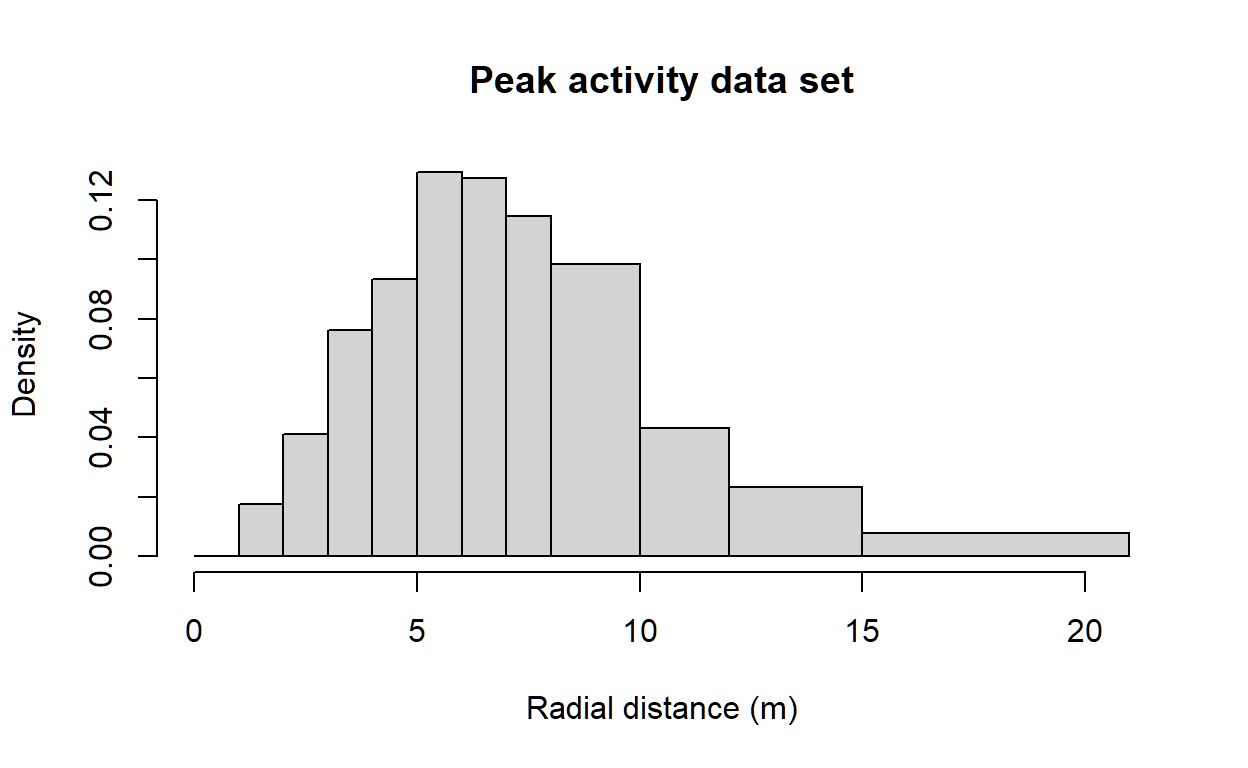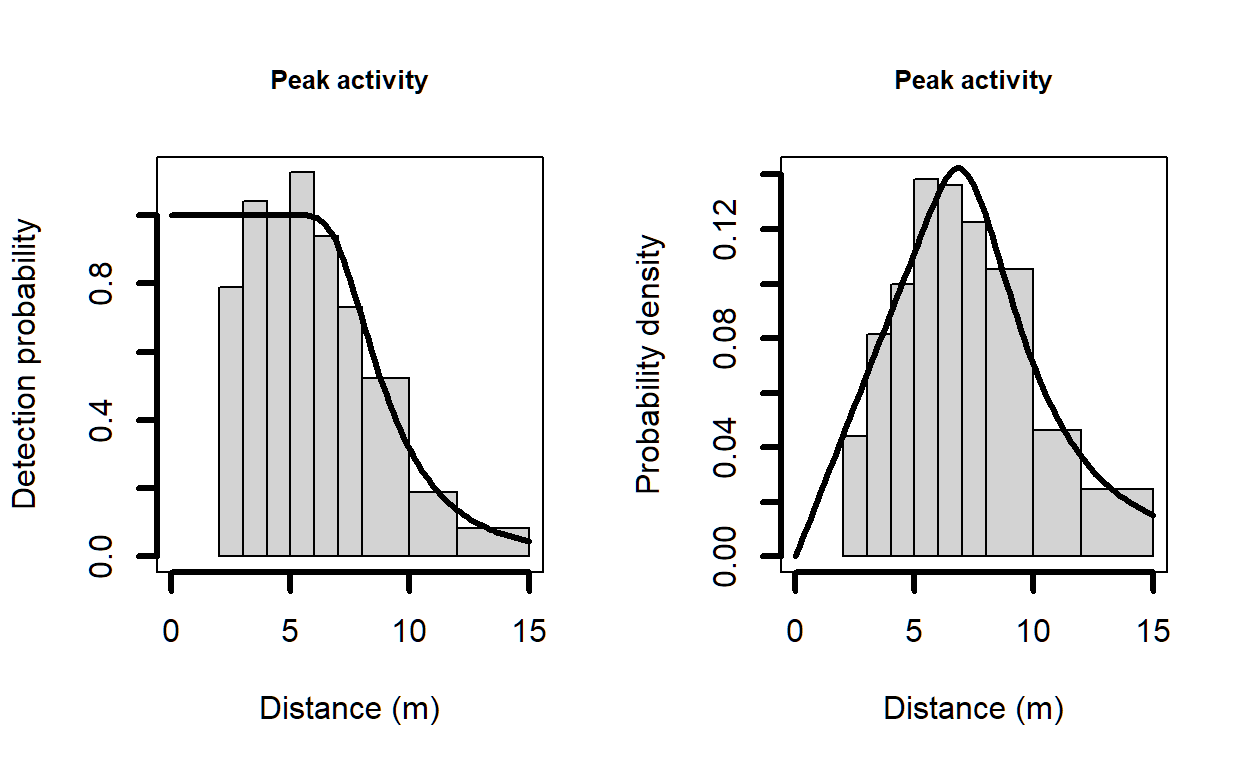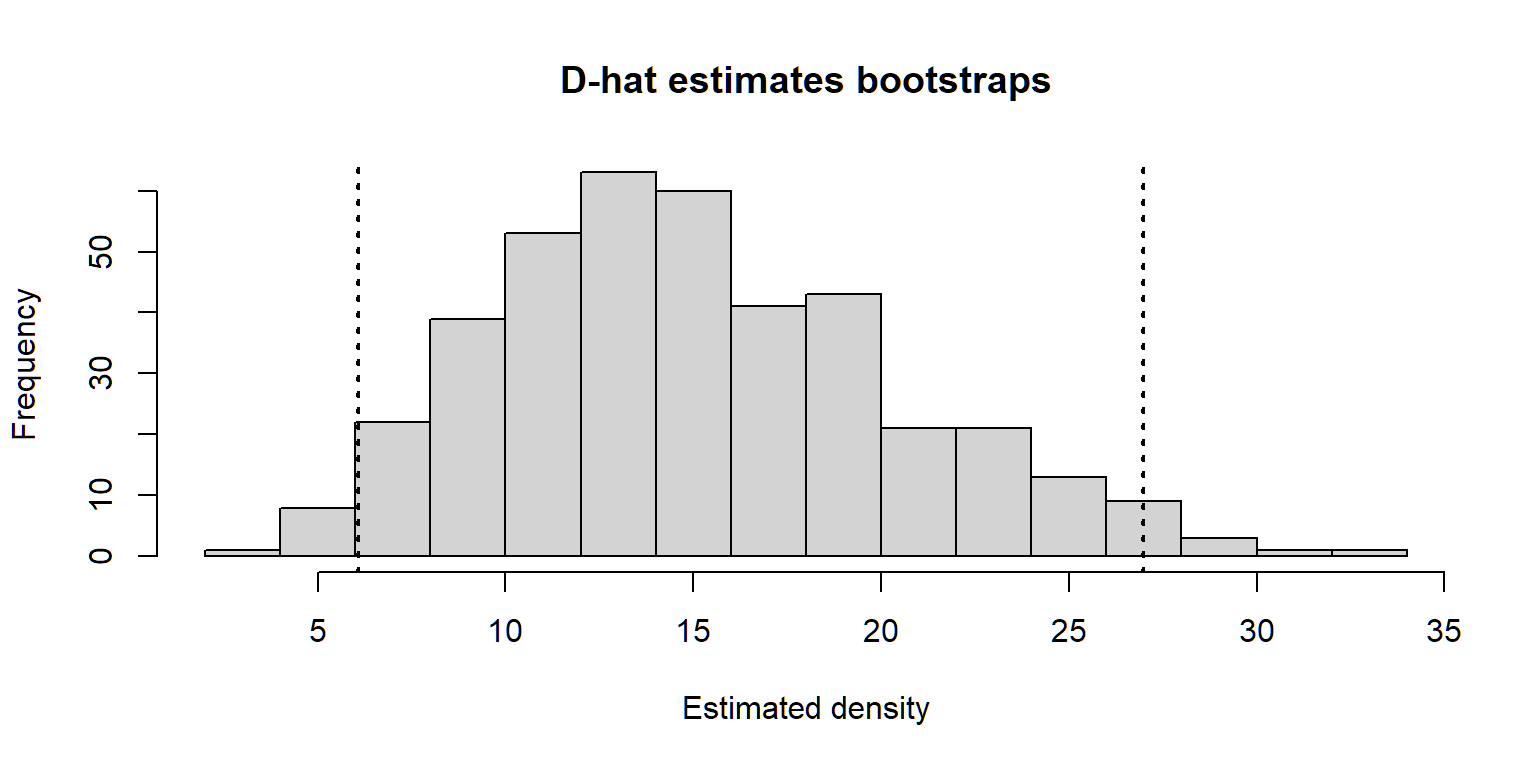# Analysis of camera trapping data

Example analysis with Ivory Coast Maxwell’s duiker.

Eric Howe and Eric Rexstad http://distancesampling.org (CREEM, Univ of St Andrews)https://creem.st-andrews.ac.uk
2020-11-24

# Analysis of camera trapping data using distance sampling

A distance sampling approach to the analysis of camera trapping data offers the potential advantage that individual animal identification is not required. However, accurate animal-to-camera detection distances are required. This requires calibration prior to the survey with images of objects of known size taken at known distances from the camera. See details in for description of the field work and data analysis. Here we present analysis of data from using the R package Distance .

## Estimating temporal availability for detection

Heat- and motion-sensitive camera traps detect only moving animals within the range of the sensor and the field of view of the camera. Animals are therefore unavailable for detection by camera traps when they are stationary, and when they are above (e.g., semi-arboreal species) or below (e.g., semi-fossorial species) the range of the sensor or the camera, regardless of their distance from the camera in two dimensions. This temporally limited availability for detection must be accounted for to avoid negative bias in estimated densities. When data are abundant, researchers may choose to include only data from times when 100% of the population can be assumed to be active within the vertical range of camera traps . However, for rarely-detected species or surveys with lower effort, it might be necessary to include most or all observations of distance. In these situations, survey duration ($$T_k$$) might be 12- or 24-hours per day, and it becomes necessary to estimate the proportion of time included in $$T_k$$ when animals were available for detection. Methods for estimating this proportion directly from CT data have been described , and it can be included in analyses to estimate density , for example as another multiplier, potentially with an associated standard errors.

## Data input

A data set for recording of detections during peak activity are included in the Distance package. Examine the DuikerCameraTraps described in the data repository .

library(Distance)
data("DuikerCameraTraps")


## Exploratory Data Analysis

A quick summary of the data set including: How many camera stations and how many detections in total.

sum(!is.na(DuikerCameraTraps$distance))   6274 table(DuikerCameraTraps$Sample.Label)


A1   A2   A3   A4   B1   B2   B3   B4   C1   C2   C3   C4   C5   C6
231   41  444  230    1 1394    8   53   30   97  486   43    1 1398
D3   D4   D5   E3   E4   E5   E6
70  117  258  102    1  375  897 

Note, three sampling stations (B1, C5, E4) had no detections. The one record for each of those stations has distance recorded as NA, but the record is important because it contains effort information.

## Distance recording

A quick examination of the distribution of detection distances; note the bespoke cutpoints causing distance bins to be narrow out to 8m, then increasing in width to the maximum detection distance of 21m.

breakpoints <- c(seq(0,8,1), 10, 12, 15, 21)
hist(DuikerCameraTraps$distance, breaks=breakpoints, main="Peak activity data set", xlab="Radial distance (m)")## Truncation decisions As described by : a paucity of observations between 1 and 2 m but not between 2 and 3 m, so we left-truncated at 2 m. Fitted detection functions and probability density functions were heavy-tailed when distances >15 m were included, so we right truncated at 15 m. # Detection function fits The conversion factor must be included either in the call to ds() or the call to bootdht(). In this vignette, it is included as an argument to bootdht(). Candidate models include the half normal key with 0 and 1 Hermite polynomial adjustment; uniform key with 1 and 2 cosine adjustments and the hazard rate key with 0, 1 and 2 cosine adjustments. conversion <- convert_units("meter", NULL, "square kilometer") trunc.list <- list(left=2, right=15) mybreaks <- c(seq(2,8,1), 10, 12, 15) hn0 <- ds(DuikerCameraTraps, transect = "point", key="hn", adjustment = NULL, cutpoints = mybreaks, truncation = trunc.list) hn1 <- ds(DuikerCameraTraps, transect = "point", key="hn", adjustment = "herm", order=2, cutpoints = mybreaks, truncation = trunc.list) uni1 <- ds(DuikerCameraTraps, transect = "point", key="unif", adjustment = "cos", order=1, cutpoints = mybreaks, truncation = trunc.list) uni2 <- ds(DuikerCameraTraps, transect = "point", key="unif", adjustment = "cos", order=c(1,2), cutpoints = mybreaks, truncation = trunc.list) hr0 <- ds(DuikerCameraTraps, transect = "point", key="hr", adjustment = NULL, cutpoints = mybreaks, truncation = trunc.list) hr1 <- ds(DuikerCameraTraps, transect = "point", key="hr", adjustment = "cos", order=2, cutpoints = mybreaks, truncation = trunc.list) hr2 <- ds(DuikerCameraTraps, transect = "point", key="hr", adjustment = "cos", order=c(2,3), cutpoints = mybreaks, truncation = trunc.list)  # Model selection adjustments from overdispersion Overdispersion causes AIC to select overly-complex models, so analysts should specify the number/order of adjustment terms manually when fitting distance sampling models to data from camera traps, rather than allowing automated selection using AIC. describes two methods for performing model selection of distance sampling models in the face of overdispersion. Here we provide R functions to perform the first of these methods. The first method of employs a two-step process. First, an overdisersion factor $$(\hat{c})$$ is computed for each key function family from the most complex model in each family. The $$\hat{c}$$ is derived from the $$\chi^2$$ goodness of fit test statistic divided by its degrees of freedom. This results in an adjusted AIC score for each model in the key function family: $QAIC = -2 \left \{ \frac{log(\mathcal{L}(\hat{\theta}))}{\hat{c}} \right \} + 2K$ Code to perform this QAIC computation is found in the function qaic.pass1: chat <- function(modobj) { # computes c-hat for a dsmodel object using Method 1 of Howe et al. (2018) test <- gof_ds(modobj) num <- test$chisquare$chi1$chisq
denom <- test$chisquare$chi1$df chat <- num/denom return(chat) } qaic <- function(modobj, chat) { # computes QAIC for a dsmodel object given a c-hat value <- 2* modobj$ddf$ds$value/chat + 2 * (length(modobj$ddf$ds$pars)+1) return(value) } qaic.pass1 <- function(...) { # Performs Pass 1 model selection based upon Method 1 of Howe et al. (2018) # Arguments are dsmodel objects; assumed all based on same key function # c-hat is computed for the most parameter-rich model in the group # qaic is calculated for each model in group based upon this c-hat # Result returned in the form of a data.frame with model name, npar, aic and qaic models <- list(...) num.models <- length(models) npar <- unlist(lapply(models, function(x) length(x$ddf$ds$par)))
modname <-  unlist(lapply(models, function(x) x$ddf$name.message))
aic <-  unlist(lapply(models, function(x) x$ddf$criterion))
chat.bigmod <- chat(models[[which.max(npar)]])
qaic <- vector(mode="numeric", length = num.models)
for (i in 1:num.models) {
qaic[i] <- qaic(models[[i]], chat.bigmod)
}
nicetab <- data.frame(modname, npar, aic, qaic)
return(nicetab)
}


Tables of QAIC values for each key function family are shown below (code for kable() calls suppressed for easier readability of results).

Table 1: QAIC values for half normal key models.
modname npar aic qaic
half-normal key function 1 25081.61 824.0688
half-normal key function with Hermite(4) adjustments 2 25021.61 826.0367
Table 2: QAIC values for uniform key models.
modname npar aic qaic
uniform key function with cosine(1) adjustments 1 24997.45 944.6180
uniform key function with cosine(1,2) adjustments 2 24995.79 946.4803
Table 3: QAIC values for hazard rate key models.
modname npar aic qaic
hazard-rate key function 2 24877.60 1510.065
hazard-rate key function with cosine(2) adjustments 3 24879.60 1516.065
hazard-rate key function with cosine(2,3) adjustments 4 24899.61 1519.157

From this first pass of model selection based on QAIC values, we find the preferable model with the hazard rate key function is one without adjustment terms. The model with the uniform key function preferred by QAIC has a single adjustment term; likewise for the half normal key function.

The second step of model selection ranks the models by their $$\hat{c}$$ values.

winners <- list(hn1, uni1, hr0)
chats <- unlist(lapply(winners, function(x) chat(x)))
modnames <- unlist(lapply(winners, function(x) x$ddf$name.message))
results <- data.frame(modnames, chats)
results.sort <- results[order(results$chats),] knitr::kable(results.sort, digits=2, row.names = FALSE, caption="Compare with Table S5 of Howe et al. (2018)")  Table 4: Compare with Table S5 of Howe et al. (2018) modnames chats hazard-rate key function 8.26 uniform key function with cosine(1) adjustments 23.22 half-normal key function with Hermite(4) adjustments 30.51 For this data set, the model chosen by this algorithm that adjusts for overdispersion is the same model (half normal key without adjustments) as would have been chosen by conventional model selection. # Sensibility check for detection parameter estimates As a check of the detection function vis-a-vis , the paper reports the effective detection radius ($$\rho$$) to be 9.4m for the peak activity data set. The effective detection radius can be derived from $$\hat{P_a}$$ as reported by the function ds as $\hat{\rho} = \sqrt{\hat{P_a} \cdot w^2}$ p_a <- hr0$ddf$fitted w <- 15 rho <- sqrt(p_a * w^2)  $$\hat{P_a}$$ is estimated to be 0.398, resulting in an estimate of $$\hat{\rho}$$ of 9.462. # Selected detection function par(mfrow=c(1,2)) plot(hr0, main="Peak activity", xlab="Distance (m)", showpoints=FALSE, lwd=3, xlim=c(0, 15)) plot(hr0, main="Peak activity", xlab="Distance (m)", pdf=TRUE, showpoints=FALSE, lwd=3, xlim=c(0, 15))## Density estimates The camera traps do not view the entire area around them, as would be the case with simple point transect sampling. The portion of the area sampled needs to be incorporated in the estimation of abundance. The data file contains a column multiplier that represents the proportion of the circle sampled. notes the camera angle of view (AOV) of 42$$^{\circ}$$. The proportion of the circle viewed is this value over 360$$^{\circ}$$. An argument to dht2 is sample_fraction, an obvious place to include this quantity. viewangle <- 42 # degrees samfrac <- viewangle / 360 conversion <- convert_units("meter", NULL, "square kilometer") peak.hr.dens <- dht2(hr0, flatfile=DuikerCameraTraps, strat_formula = ~1, sample_fraction = samfrac, er_est = "P2", convert_units = conversion) print(peak.hr.dens, report="density")  Summary statistics: .Label Area CoveredArea Effort n k ER se.ER cv.ER Total 40.37 1015.748 12317058 5865 21 0 0 0.302 Density estimates: .Label Estimate se cv LCI UCI df Total 14.5106 4.384 0.302 7.8351 26.8734 20.094 Component percentages of variance: .Label Detection ER Total 0.23 99.77 # Bootstrap for variance estimation To produce a more reliable estimate of the precision of the point estimate, produce bootstrap estimates using bootdht. Note, with an Intel i5 generation processor, this call to bootdht required ~3hrs of computing; you may desire more bootstrap replicates than provided here. Two issues to note when using bootdht with these camera trap data: • because of the viewing angle of the camera, we must specify the sample_fraction argument just as was done in the call to dht2 • in , estimates of density rather than estimates of abundance are presented. To produce estimates from bootdht consistent with , we write our own simple summary function to extract density estimates from each bootstrap replicate. This is instead of relying upon the default function bootdht_Nhat_summarize provided in the Distance package, which produces estimates of abundance rather than density. viewangle <- 42 # degrees samfrac <- viewangle / 360 mysummary <- function(ests, fit){ return(data.frame(Dhat = ests$individuals$D$Estimate))
}
duiker.boot.hr <- bootdht(model=hr0, flatfile=DuikerCameraTraps, resample_transects = TRUE,
nboot=400, summary_fun=mysummary, sample_fraction = samfrac,
convert.units = conversion)


Confidence limits computed via the percentile method of the bootstrap.

print(summary(duiker.boot.hr))

Bootstrap results

Boostraps          : 400
Successes          : 399
Failures           : 1

median  mean  se  lcl   ucl   cv
Dhat  14.27 15.06 5.4 6.08 26.98 0.38
hist(duiker.boot.hr$Dhat, breaks = 20, xlab="Estimated density", main="D-hat estimates bootstraps") abline(v=quantile(duiker.boot.hr$Dhat, probs = c(0.025,0.975), na.rm=TRUE), lwd=2, lty=3)Figure 1: Distribution of density estimates from bootstrap replicates.

Note the confidence limits computed from the bootstrap are somewhat wider than the confidence limits computed via dht2.

Bessone, M., Kühl, H. S., Hohmann, G., Herbinger, I., N’Goran, K. P., Asanzi, P., … Fruth, B. (2020). Drawn out of the shadows: Surveying secretive forest species with camera trap distance sampling. Journal of Applied Ecology, 57(5), 963–974. https://doi.org/10.1111/1365-2664.13602
Howe, E. J., Buckland, S. T., Després-Einspenner, M.-L., & Kühl, H. S. (2017). Distance sampling with camera traps. Methods in Ecology and Evolution, 8(11), 1558–1565. https://doi.org/10.1111/2041-210X.12790
Howe, E. J., Buckland, S. T., Després-Einspenner, M.-L., & Kühl, H. S. (2019). Model selection with overdispersed distance sampling data. Methods in Ecology and Evolution, 10(1), 38–47. https://doi.org/10.1111/2041-210X.13082
Howe, E. J., Buckland, S. T., Després-Einspenner, M.-L., Kühl, H. S., & Buckland, S. T. (2018). Data from: Distance sampling with camera traps. https://doi.org/https://doi.org/10.5061/dryad.b4c70
Miller, D., Rexstad, E., Thomas, L., Marshall, L., & Laake, J. (2019). Distance sampling in r. Journal of Statistical Software, Articles, 89(1), 1–28. https://doi.org/10.18637/jss.v089.i01
Rowcliffe, J. M., Kays, R., Kranstauber, B., Carbone, C., & Jansen, P. A. (2014). Quantifying levels of animal activity using camera trap data. Methods in Ecology and Evolution, 5(11), 1170–1179. https://doi.org/10.1111/2041-210X.12278